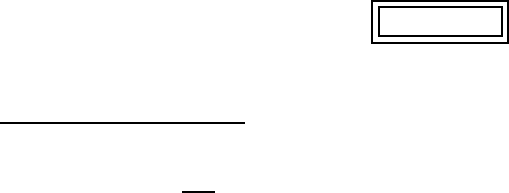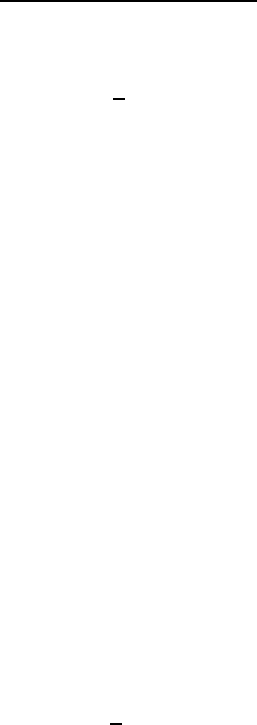# 4-11f-test3

22 views8 pages
School
Course
ProfessorEGR 265, TEST III 1
EGR 265, Math Tools for Engineering Problem Solving
November 30, 2011, 50 minutes
Name: . . . . . . . . . . . . . . . . . . . . . . . . . . . . . . . . . . . . . . . . . .
TEST III
Problem 1 (9+9 points)
(a) Let f(x, y) = 2x
xy. Find fxx +fyy.
(b) For the function g(x, y) = xln(x2+y) ﬁnd gx,gyand gxy.
Unlock document

This preview shows pages 1-3 of the document.
Unlock all 8 pages and 3 million more documents.EGR 265, TEST III 2
Problem 2 (9+9 points)
(a) For the function h(x, y) = e(x4+y2)ﬁnd its direction and rate of steepest descent at
the point P(1,2).
(b) Find the directional derivative of h(x, y) at P(1,2) in the direction of the vector
from P(1,2) to Q(4,2).
Unlock document

This preview shows pages 1-3 of the document.
Unlock all 8 pages and 3 million more documents.EGR 265, TEST III 3
Problem 3 (12+6 points)
(a) Find an equation for the tangent plane to the graph of z=xy + cos(xy) + 1 at
the point (π
2,0,1).
(b) Also, ﬁnd parametric equations for the normal line to the graph of z=xy +cos(x
y) + 1 at (π
2,0,1).
Unlock document

This preview shows pages 1-3 of the document.
Unlock all 8 pages and 3 million more documents.